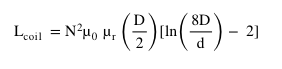# Coil Inductance Calculator

 Relative permeability (μr) No. of turns Coil Radius MetersCentimetersMillimetersInches Solenoid Length MetersCentimetersMillimetersInches

### OUTPUT

 Inductance HmHµH

This calculator is designed to calculate the inductance of the coil, given the Relative permeability, No. of turns, Coil Radius and Solenoid Length.

Inductance is the property of a component (in our case coil) that opposes the change of current flow through it. Inductance does not oppose the current but it opposes the change in current flow through the circuit. When electrical current changes its flows through the coil, it produces an electromagnetic field in the wire that opposes the current flow. The symbol for inductance is the Henry and the unit is H. Inductance in a coil is directly proportional to ‘Number of Turns’, ‘Area’ and inversely proportional to the ‘Length of coil’.

EquationWhere:

Lcoil = Inductance of the coil in henries (H)

N2 = Number of turns

u0 = Permeability of free space

ur = Relative permeability

D = Loop diameter

d = Wire diameter

There are two ways in which inductance is used:

Self-inductance:   Self-inductance is the property of a circuit, often a coil, in which a change in current in a circuit produces an induced electro-motive-force in the same circuit, in other words it is an inductance, typically within a single coil.

Mutual-inductance:   Mutual inductance is an inductive effect where a change in current in one circuit causes a change in voltage across a second circuit as a result of a magnetic field that links both circuits. This effect is used in transformers.